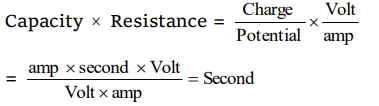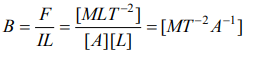## Units and Measurement Questions and Answers Part-19

1. Let $\left(\epsilon_{0}\right)$ denotes the dimensional formula of the permittivity of the vacuum and $\left(\mu_{0}\right)$ that of the permeability of the vacuum. If M = mass, L = lenth, T = time and l = electric current, then
a) $\left[\epsilon_{0}\right]=M^{-1}L^{-3}T^{2}I$
b) $\left[\epsilon_{0}\right]=M^{-1}L^{-3}T^{4}I^{2}$
c) $\left[\mu_{0}\right]=MLT^{-2}I^{-2}$
d) Both b and c

Explanation: Both b and c

2. Dimensions of CR are those of
a) Frequency
b) Energy
c) Time period
d) Current

Explanation:3. The physical quantity that has no dimensions
a) Angular Velocity
b) Linear momentum
c) Angular momentum
d) Strain

Explanation: Strain has no dimensions

4. $ML^{-1}T^{-2}$  represents
a) Stress
b) Young's Modulus
c) Pressure
d) All the above three quantities

Explanation: All the above three quantities

5. Dimensions of magnetic field intensity is
a) $M^{0}L^{-1}T^{0}A^{1}$
b) $MLT^{-1}A^{-1}$
c) $ML^{0}T^{-2}A^{-1}$
d) $MLT^{-2}A$

Explanation:6. The force F on a sphere of radius $'a'$ moving in a medium with velocity 'v' is given by $F=6\pi\eta av$  . The dimensions of $\eta$ are
a) $ML^{-1}T^{-1}$
b) $MT^{-1}$
c) $MLT^{-2}$
d) $ML^{-3}$

Explanation:7. Which physical quantities have the same dimension
a) Couple of force and work
b) Force and power
c) Latent heat and specific heat
d) Work and power

Explanation:8. Two quantities A and B have different dimensions. Which mathematical operation given below is physically meaningful
a) $A\diagup B$
b) $A+ B$
c) $A- B$
d) None

Explanation: Quantities having different dimensions can only be divided or multiplied but they cannot be added or subtracted.

9. The physical quantity which has the dimensional formula $M^{1}T^{-3}$  is
a) Surface tension
b) Solar constant
c) Density
d) Compressibility10. A force F is given by $F=at+bt^{2}$   where t is time. What are the dimensions of a and b
a) $MLT^{-3}$  and $ML^{2}T^{-4}$
b) $MLT^{-3}$  and $MLT^{-4}$
c) $MLT^{-1}$  and $MLT^{0}$
d) $MLT^{-4}$  and $MLT^{1}$